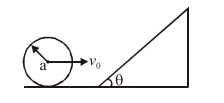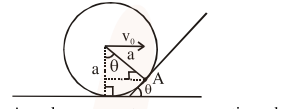# A sphere of radius ' a ' and mass ' m ' rolls along`
Question:

A sphere of radius ' $a$ ' and mass ' $m$ ' rolls along a horizontal plane with constant speed $v_{0}$. It encounters an inclined plane at angle $\theta$ and climbs upward. Assuming that it rolls without slipping, how far up the sphere will travel ?1. $\frac{10 v_{0}^{2}}{7 g \sin \theta}$

2.  $\frac{v_{0}^{2}}{5 g \sin \theta}$

3. $\frac{2}{5} \frac{v_{0}^{2}}{g \sin \theta}$

4. $\frac{v_{0}^{2}}{2 g \sin \theta}$

Correct Option: 1

Solution:$\mathrm{mv}_{0} \mathrm{a} \cos \theta+\frac{2}{5} \mathrm{ma}^{2} \omega$

$=m v a+\frac{2}{5} m a^{2} \omega^{1}$

$\mathrm{mv}_{0} \mathrm{a}\left[\frac{2}{5}+\cos \theta\right]=\frac{7}{5} \mathrm{mva}$

$\mathrm{v}=\frac{5}{7}=\mathrm{v}_{0}\left[\frac{2}{5}+\cos \theta\right]$

$\frac{1}{2} \mathrm{mv}^{2}+\frac{1}{2} \mathrm{I} \omega^{2}=\frac{7}{10} \mathrm{mv}^{2}=\mathrm{mgh}$

No option Maching